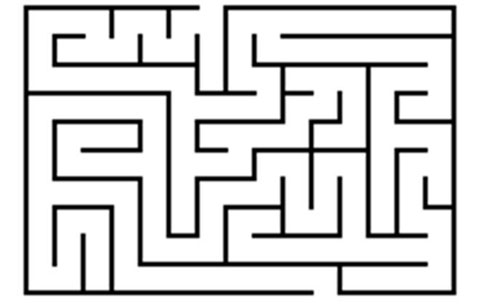# e-maje-in

## Problem:-

Ankit is in maze. The command center sent him a string which decodes to come out from the maze. He is initially at (0, 0). String contains L, R, U, D denoting left, right, up and down. In each command he will traverse 1 unit distance in the respective direction.For example if he is at (2, 0) and the command is L he will go to (1, 0).
Input:
Input contains a single string.
Output:
Print the final point where he came out.
Constraints:
1 ≤ |S| ≤ 200
SAMPLE INPUT

`LLRDDR`
SAMPLE OUTPUT

`0 -2`
Explanation

None
Time Limit:1.0 sec(s) for each input file.
Memory Limit:256 MB
Source Limit:1024 KB

## solution:-

#include<string.h>
void main()
{
int i,l,x=0,y=0,a;
char s;
scanf(“%s”,s);
l=strlen(s);
for(i=0;i<=l1;i++)
{
a=s[i];
if(a==76)
x=x1;
if(a==82)
x=x+1;
if(a==68)
y=y1;
if(a==85)
y=y+1;
}
printf(“%d %d”,x,y);
}